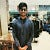# Linear Algebra for QC

a vector space is a set of vectors which follow certain axioms:

the operations a vector space consists are vector addition and scalar multiplication(remember this ,since it will make it easier to understand Hilbert space later).

linear independence: lets consider a set of vectors a,b,c,…,k

now lets consider the set of all linear combinations of these vectors . c1a+c2b+c3c+…..ckk , where c1,c2,c3…,ck are scalars.

if any of these linear combinations results in a 0 vector and if all the scalars are also 0 then such a collection of vectors are linearly independent.

linearly dependent: if the linear combination of the…

# Quantum computer vs classical computer(Superposition)

What makes a Quantum computer different from a classical computer?

To answer this we need to understand the basic unit of data in both. a classical computer consists of a ‘bit’ as a unit of computation. whereas a quantum computer consists of what is called a ‘qubit’. there are 2 states a bit can assume 0 and 1 namely. to understand the states of a qubit, lets consider a thought experiment. lets say there is a cat in a box along with a mechanism to kill it . the only catch is that this mechanism is uncertain, it has some…

Probability for QC

I am assuming that you are familiar with the basic concepts of set theory/notation and probability theory. so lets start where the rigor of probability begins to glimpse.

Random variables- although we call them variables ,its actually a function/mapping of the sample space to real numbers. this means that events are mapped to either discrete values(giving rise to discrete random variables) or continuous values. mathematically it could be written as:

let Ω be the sample space.

let X be the random variable.

let R be the set of real numbers.

then this mapping would be written as…

# Complex conjugate transpose

Lets talk about inner product of 2 vectors first.

lets say we have 2 column matrices ,i.e vectors, a and b (written in bold). <a,b>=(a^⊤)(b) ,this is the definition of inner product.

for example if :a=

then a^⊤ would be:

# Complex numbers for QC

I am assuming that you are familiar with basic Trignometry and concepts of vectors .I will also assume that you are familiar with basics of complex no.

so we are going to start with euler representation of complex no. using complex exponentials.

We know that complex no. exist in a plane called the complex plane that is made of a real axis and an imaginary axis which are orthogonal to each other. …

# Quantum Computing

This medium account is dedicated to give casual readers an in depth understanding of quantum computation and the necessary quantum mechanics. we will cover the necessary Mathematics and physics to really get a sense of working with computation in the quantum system. this is an attempt to get people an equivalent of a first course in quantum computing being taught in universities for undergrad and postgrad students for free. the only prerequisite would be mathematical maturity , and an excitement towards the field, rest would be up to me. so lets get started. stay tuned for the next blog where we will begin our first lecture.